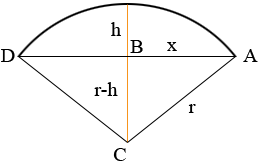Subject: arc Name: Thomas Who are you: Student what is the height of the arc of the earth over a certain distance? Hi Thomas, I drew an arc of a circle and labeled some points and distances.In my diagram r is the radius of the Earth, the distance from D to A is 2x, B is the midpoint of the line section DA and h is the unknown height. I haven't included units, you can use whatever units you wish as long as you use the same units for each of the distances. The triangle ABC is a right triangle and hence, by Pythagoras' Theorem r2 = x2 + (r-h)2 which simplifies to h2 - 2rh + x2 = 0 Solve for h. Penny